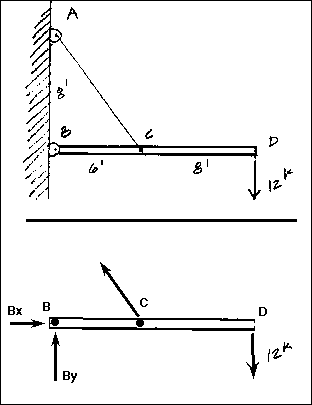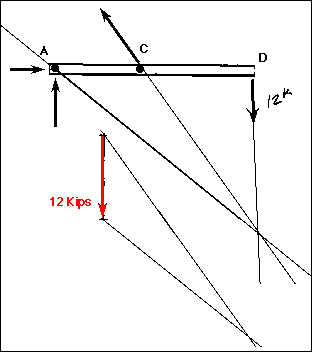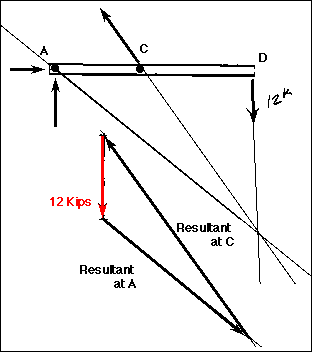## Lecture 15 Example Problem

Three-Force MembersGiven:
Determine:
a. the FBD of the horizontal element using the images below
b. the reactions at B and C algebraically
c. the reactions at B anc C graphically

Solution:
a. The free body diagram of the horizontal member will include the 12k force at D and the reactions at B and C. The pinned support at B will have a horizontal and a vertical component although the angle at which the total force B acts is unknown at this point. The direction of C is already known because it must act in the direction of the wire. Hoever, it is more easily solved when it is broken into rectangular components.

b. Apply the familiar equations of static equilibrium to algebraicly solve for the reactions at B and C.

Sum Fx = 0 = Bx + Cx
Sum Fy = 0 = -12k + By + Cy
Sum MB = 0 = -Cy(6ft) + 12k(14ft)
Solve for Cy using the moment equation:
6Cy = 168kft
Cy = 28k
Solve for By using Cy in Sum Fy = 0:
-By = -12k + 28k
-By = 16k
By = -16k
Now there are two more unknowns, Bx and Cx, but only one other equation. However, it can be seen that C is part of a 3-4-5 triangle. Using this information, solve for C and Cx:
Cy = 28k = 4/5 C
C = 35k
Cx = -3/5 (35k)
Cx = -21k
Now solve for Bx:
Bx = -Cx
Bx = 21k
Finally, solve for B using the Pythagorean Theorem:
B = SQRT (21k^2 + (-16k)^2) = SQRT (256) = 16k acting down and to the right
The angle for the reaction at B would be:
ĝ = arctan (-16 / 21)
ĝ = 37.3 degrees below the x axisc. Graphic Solution.

Remember that in order for an element in a three-force system to remain in equilibrium, any one force must be the resultant of the other two forces. Additionally, if the system is in equilibrium and if the forces are not parallel, they must be concurrent.

Now, extend the lines of action of the two forces that have a known direction until they intersect. This is the point of origin for the three-force system. It is a VERY important point! Now, the line of action and the direction of the reaction at B can be found by simply drawing a vector from point B to the point of origin. This is the line of action of the reaction force. The direction of the resulting third force is also known when inspection determines the kind of force that must be applied to put the system into equilibrium. The magnitude of B and C can be found using the triangle method and making assumptions about the sense of the forces.Copyright İ 1995, 1996 by Chris H. Luebkeman and Donald Peting
Copyright İ 1997 by Chris H. Luebkeman